<![CDATA[Math Is Fun Forum / Simple groups]]> 2009-06-20T11:56:45Z FluxBB http://www.mathisfunforum.com/viewtopic.php?id=10734 <![CDATA[Re: Simple groups]]> You're certainly on the right track.  But when you have that the intersection contains all of P, and hence all of Q, you actually get that P and Q are the same exact two subgroups.  Since we started out with two different subgroups, obviously this can't happen.

]]>
http://www.mathisfunforum.com/profile.php?id=2143 2009-06-20T11:56:45Z http://www.mathisfunforum.com/viewtopic.php?pid=113930#p113930
<![CDATA[Re: Simple groups]]> Thank you! the intersection can't be all of P because in that case the intersection of P and Q must be P, this is, P C Q and that it's a contradiction since P is a Sylow P-subgroup a maximal p-soubgroup.......?????????????

]]>
http://www.mathisfunforum.com/profile.php?id=38381 2009-06-19T14:27:54Z http://www.mathisfunforum.com/viewtopic.php?pid=113850#p113850
<![CDATA[Re: Simple groups]]> In a group G of order 56, the Sylow 7-subgroups have order 7.  Now let's assume we have two Sylow subgroups, P and Q.  Their intersection, call it I, has to be not only a subgroup of G, but quite clearly a subgroup of P as well.  By Lagrange's theorem, the order of I must divide the order of P.  But P's order is 7.  So either we have that the order of I is 1 (the identity) or 7 (all of P).

I'll leave it to you to explain why the order of I can't be 7.

]]>
http://www.mathisfunforum.com/profile.php?id=2143 2009-06-08T21:26:33Z http://www.mathisfunforum.com/viewtopic.php?pid=113138#p113138
<![CDATA[Re: Simple groups]]> I have a question for JaneFairfax or anyone who want it to answer, in the #22 in the case that the order of G is 56 why the union of the the Sylow 7-subgroups has 48 non-identity elements? i mean why the intersection is only the indentity? sorry maybe that is very easy to see but im not quite familiar with this definitions..

]]>
http://www.mathisfunforum.com/profile.php?id=38381 2009-06-08T21:15:21Z http://www.mathisfunforum.com/viewtopic.php?pid=113137#p113137
<![CDATA[Re: Simple groups]]>

I didnt know what counted as heavy machinery.

Heavy machinery to me would be the theorem of Burnside and FeitThompson.  With those, the task becomes rather trivial.

So, now that we have completed this assignment, whats next?

I'd be impressed if we did all groups of order up to 1000.

]]>
http://www.mathisfunforum.com/profile.php?id=2143 2009-05-16T20:12:32Z http://www.mathisfunforum.com/viewtopic.php?pid=112087#p112087
<![CDATA[Re: Simple groups]]> Yeah, I wasnt sure if I should really use the simplicity of the alternating groups. I didnt know what counted as heavy machinery. You are obviously accustomed to a lot of heavy-duty stuff in group theory whereas I am only picking up from where I left off years ago, so what may be tough going for me may be feather light for you.So, now that we have completed this assignment, whats next?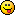]]>
http://www.mathisfunforum.com/profile.php?id=6777 2009-05-16T18:35:54Z http://www.mathisfunforum.com/viewtopic.php?pid=112078#p112078
<![CDATA[Re: Simple groups]]> Now that you're done, the theorem I was speaking of was:

If G is a simple group, and H is a subgroup with [G : H] = n, then there exists an injective homomorphism

.  That is, G may be identified with a subgroup of A_n.

Using this, here is how I did it.  First I proved this lemma:

Let p be a prime, and |G| = p^k * m, where it may be that p | m.  If |G| does not divide m!/2, then G is not simple.

Now using 120 as an upper bound for |G|, this may be now used to conclude statements:

If 2^4 divides |G| and |G| < 120, then G is not simple.
If 3^3 divides |G| and |G| < 120, then G is not simple.
If 5^2 divides |G| and |G| < 120, then G is not simple.
If 11 divides |G| and |G| < 120, then G is not simple.
If 13 divides |G| and |G| < 120, then G is not simple.
If 17 divides |G| and |G| < 120, then G is not simple.
If 19 divides |G| and |G| < 120, then G is not simple.

These divisibility conditions will get 22 group orders.  We can squeeze more out of it if we forgo the use of the upper bound and just use a particular order.  This will get groups of order 12, 18, 20, 24, 28, 36, 40, 42, 45, 74, 92, 98, 116.  Each argument is just finding the right p^k so that |G| = p^k * m where p^k does not divide m!/2.

Most of the rest are prime powered or groups of order pq (with p ≠ q), all of which of course not simple.  This only left 8 groups which were rather manageable.  On each of these, either the counting arguments or a direct application of the theorem I gave above worked.  That is, on all except 90.  Here was my proof for 90:

Let G be a group of order 90, and assume G is simple.  The number of Sylow 5-subgroups must divide 90/5 = 18, and is congruent to 1 modulo 5.  As G is simple, we conclude this number must be 6.  Let H be a Sylow 5-subgroup, and so [G : N(H)] = 6.  Thus, we may identify G as a subgroup of A_6.  But now |A_6| = 360, |G| = 90, and so [A_6, G] = 4, with A_6 being a simple subgroup.  Thus, A_6 may be identified with a subgroup of A_4, a contradiction.

]]>
http://www.mathisfunforum.com/profile.php?id=2143 2009-05-16T16:43:28Z http://www.mathisfunforum.com/viewtopic.php?pid=112077#p112077
<![CDATA[Re: Simple groups]]> And now, how about a bonus extra?Ive just seen two proofs of this on the Web. Here is my own adaptation of them. Note that it assumes the knowledge that

is simple.

Suppose there is a simple group

of order 120. The number of Sylow 5-subgroups must be 6. If
is one of them, then
and so there is a homomorphism
with
. (Indeed
is none other than the
in my oft-quoted Humphreys corollary.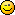) As
is simple,
. Hence
is injective and so there is a simple subgroup
of
of order 120.

As

, we have
and so by simplicity of
either
or
. The former is impossible as it would imply that the number of elements of the form
for
would be
 way above the order of
.Thus
. Then
and so there is a homomorphism
with
. By simplicity of
,
meaning that
is injective and leading to the utter balderdash that
is isomorphic to a subgroup of
.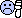cannot be simple.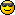In fact, this method can also be used to show that groups of order 90 are not simple as well. However, the method I used has the advantage of not assuming that the alternating group of degree 6 is simple.

The fact that

is simple for
is established in several stages. First it is shown that that
is simple. After a couple of other results are proved, the job is completed using mathematical induction. The proof of the simplicity of
is readably done in not more that two pages by Humphreys. Humphreys first demonstrates that
has precisely five conjugacy classes, one of which is the singleton class containing just the identity. Any normal subgroup of
would have to be a union of this trivial conjugacy class with none, some or all of the other conjugacy classes. By determining the sizes of all the conjugacy classes, Humphreys is able to show that the number of elements in any union of the trivial class with some but not all of the other classes cannot be a divisor of 60, thus demonstrating by Lagrange that
cannot have any nontrivial proper normal subgroup.]]>
http://www.mathisfunforum.com/profile.php?id=6777 2009-05-16T14:28:54Z http://www.mathisfunforum.com/viewtopic.php?pid=112071#p112071
<![CDATA[Re: Simple groups]]> And finally:There are 1 or 7 Sylow 2-subgroups. If 7, the union of all the Sylow 2-subgroups contains at most

elements. If this be the case, the remaining 6 elements together with the identity must make up the unique Sylow 7-subgroup. Otherwise let
and
be distinct Sylow 2-subgroups. Then
and so
has more than 16 elements. As
must have order divisible by 16 and dividing 112, this means
. If
then
has index 2 in
and
, so is normal in
and
and hence
.

This leaves

. Suppose all the 7 Sylow 2-subgroups have pairwise intersections of 4 elements. Then the minimum number of elements in their union is when the same 4 elements are in all their intersections, which would be
. This would mean there could not be 8 Sylow 7-subgroups and so the Sylow 7-subgroup must be normal.

Whee! I did it!Well, at least I hope I have.]]>
http://www.mathisfunforum.com/profile.php?id=6777 2009-05-16T10:40:59Z http://www.mathisfunforum.com/viewtopic.php?pid=112060#p112060
<![CDATA[Re: Simple groups]]>Such a group

has 1 or 4 Sylow 3-subgroups, each of order 9. Suppose 4 and let
be one of them. Then
and so by Humphreyss corollary, there is a normal subgroup
of
contained in
such that 4 divides
and
divides 4! = 24.
showing that
is not simple.

Wow, it really is simple when you know how.]]>
http://www.mathisfunforum.com/profile.php?id=6777 2009-05-16T10:11:37Z http://www.mathisfunforum.com/viewtopic.php?pid=112059#p112059
<![CDATA[Re: Simple groups]]>Okay, this trick is adapted from something Ive just noticed on p.161 of Humphreys, which is devoted to a much different problem (namely the proving that every group of order 36 is soluble).

There are 1 or 10 Sylow 3-subgroups, each of order 9. If 10, the union of all the Sylow 3-subgroups contains at most

elements. If there are really that many, then there cannot be 6 Sylow 5-subgroups, so the Sylow 5-subgroup in this case is normal. Otherwise there exist distinct Sylow 3-subgroups
and
with a nontrivial intersection. It follows that
. The set
therefore contains
elements. So
, the subgroup generated by
and
, must contain at least 27 elements. Its order also must be divisible by 9 and must divide 90. Hence
. If 45, then
is a normal subgroup, being of index 2. Otherwise, it is the whole group
; then I claim that
is a normal subgroup of
. For
and
are both Abelian (see post #10 above) and so
is normal in both of them; hence
must be normal in the subgroup generated by
and
.

Whew! That took a while to get through.

]]>
http://www.mathisfunforum.com/profile.php?id=6777 2009-05-16T08:57:21Z http://www.mathisfunforum.com/viewtopic.php?pid=112058#p112058
<![CDATA[Re: Simple groups]]> If you want some help, there is a theorem which is quite useful for both Sylow theory and in general.  I've scanned (briefly) through what I could get my hands on in Humphrey, but could not find this theorem.

]]>
http://www.mathisfunforum.com/profile.php?id=2143 2009-05-12T21:31:21Z http://www.mathisfunforum.com/viewtopic.php?pid=111758#p111758
<![CDATA[Re: Simple groups]]> Oops, I did it again for 90. Oh well, back to square one.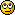I only have three left: 72, 90, 112. Man, these are tough!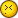]]>
http://www.mathisfunforum.com/profile.php?id=6777 2009-05-12T18:12:59Z http://www.mathisfunforum.com/viewtopic.php?pid=111735#p111735
<![CDATA[Re: Simple groups]]> Post #22 has the typo

But is fine otherwise.

]]>
http://www.mathisfunforum.com/profile.php?id=2143 2009-05-12T15:36:25Z http://www.mathisfunforum.com/viewtopic.php?pid=111732#p111732
<![CDATA[Re: Simple groups]]> From post #20

For 90, the Sylow 3-subgroup is unique.

You can not conclude this from direct application of the Sylow theorems.  There could be 10 Sylow 3-subgroups.  Even if you could, simply stating this is not sufficient.

Also, whenever you post numbers where prime factorization is important, you should also post the factorization.  Instead of just writing 90, write

]]>
http://www.mathisfunforum.com/profile.php?id=2143 2009-05-12T15:24:17Z http://www.mathisfunforum.com/viewtopic.php?pid=111730#p111730